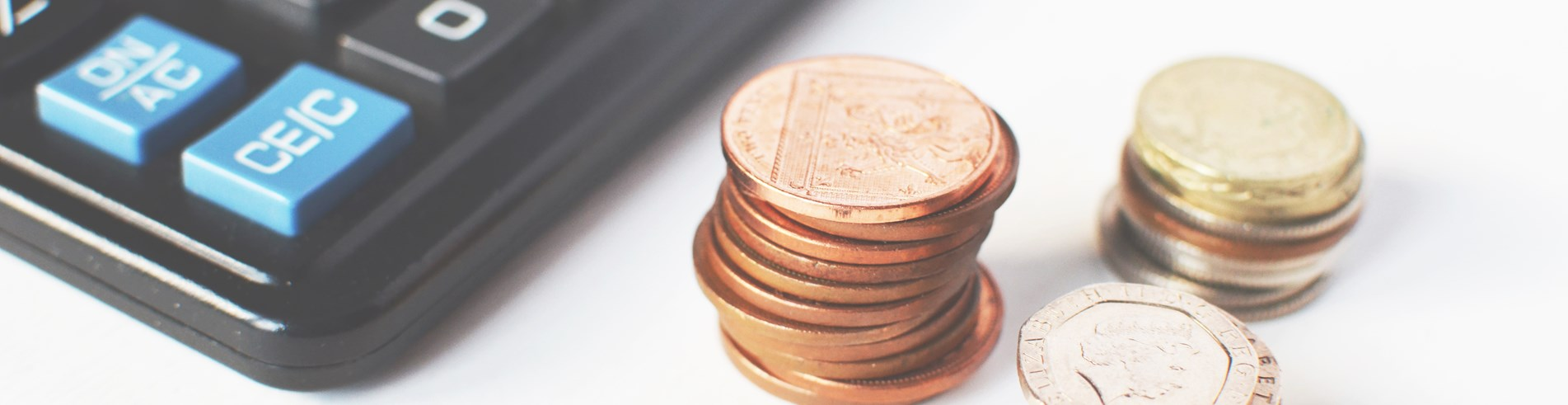Close# Maths

Mathematics is a core subject and as such is studied by every student in the school from Year 7 to Year 11. Maths is an all embracing and pervading part of life, helping us to create, plan, organise and solve problems.

Mathematics is the universal language of our environment, helping mankind explain and create within it for thousands of years. From playing games to playing music, maths is vital to helping students fine tune their creativity and turn their dreams into reality.

Our curriculum is based on the national programmes of study and lessons are accessible to all students in the class to create opportunities and build familiarity and accuracy in techniques and allow students to use their skills in everyday life. We cover a variety of topics that allow students to excel in their learning in different fields. Students know that when they come to lessons, they will be entering a positive environment for learning where they learn new techniques and challenges are accepted.

We revisit topics throughout KS3 and 4 to consolidate understanding and encourage students to link their work with other areas to extend their thinking. We have a strong focus on developing problem-solving skills alongside mathematical content to ensure we develop resilient, independent learners ready to tackle GCSE and A-level maths.

##### CURRICULUM INFORMATION

 Year Autumn Spring Summer 7 Number and calculations Factors and multiples Area and volume Fractions Expressions Fractions, decimals and percentages Charts and averages Shapes and angles Ratio Equations Equations Sequences Graphs 8 Number Area and volume Expressions Fractions, decimals and percentages Probability Equations Shapes and angles Ratio Pythagoras and trigonometry Graphs Sequences Charts and averages Transformations 9 Factors and multiples Indices and standard form Expressions and equations Charts and averages Area and volume Fractions, decimals and percentages Ratio Shapes and angles Surds Pythagoras’ theorem Right angled Trigonometry Probability 10 Indices and standard form Linear and quadratic expressions and equations Charts and averages Cumulative frequency Transformations Area and volume Fractions, decimals and percentages Linear graphs Constructions Simultaneous equations Ratio and proportion Shape and angles Circle theorems Graphs of other functions Pythagoras’ theorem Right angled and non-right-angled trigonometry 11 Non-right-angled trigonometry Algebraic manipulation Quadratic equations   Functions Algebraic proof Vectors Surds Ratio and proportion Linear and quadratic graphs Cumulative frequency Circle theorems Linear and quadratic simultaneous equations
Maths is very useful as it teaches practical and logical thinking which can be applied to any problem in the future.
Year 10 student
Maths is a great lesson that really gets you thinking. I really enjoy expanding my mathematical skills.
Year 9 student